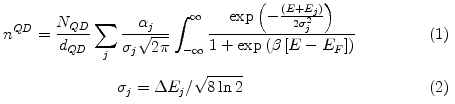nextnano.com  nextnano³  Download | Search | Copyright | Publications  * password protected nextnano³ softwarequantum-dot-layer-density.htm

# Quantum-dot-layer-density

Special density model for layers of quantum dots: In dot layers the density of states depends first on the electronic structure of the individual dots and second on the density and thickness of the layer. This command should emulate a dot layer with dots of a certain energy distribution due to a natural size fluctuation of the dots.

1D-only !!!The density is calculated by summing over all eigenstates with an artificial line-broadening of gaussian shape. The line width sigmaj (`cb-width-eigenvalues)`has to be provided by the user as well as the degeneracy alphaj (`cb-degeneracy-eigenvalues)`. To determine the charge density one also needs the density of quantum dots within the layer NQD (`sheet-density)` and the thickness of the layer dQD (`sheet-width)`

```!-----------------------------------------------------------------! \$quantum-dot-layer-density                               optional !  density-number                           integer        required !  quantum-region                           integer        optional !  model-active                             character      optional !  use-bound-states                         character      optional !  sheet-density                            double         optional !  sheet-width                              double         optional !  cb-set-number                            integer        optional !  cb-number-of-eigenvalues                 integer        optional !  cb-eigenvalues                           double_array   optional !  cb-width-eigenvalues                     double_array   optional !  cb-degeneracy-eigenvalues                integer_array  optional !  cb-occupy-levels                         integer_array  optional !  vb-set-number                            integer        optional !  vb-number-of-eigenvalues                 integer        optional !  vb-eigenvalues                           double_array   optional !  vb-width-eigenvalues                     double_array   optional !  vb-degeneracy-eigenvalues                integer_array  optional !  vb-occupy-levels                         integer_array  optional ! \$end_quantum-dot-layer-density                           optional ! !-----------------------------------------------------------------! ```

## Synatx:

`density-number = 1`

Sequencial numbering for several dot layers

`quantum-region = 1`

A quantum dot layer is always located in a quantum region, therefore the number of this region has to specified.

`model-active = yes / no`

Flag whether this dot-layer-density is active or not.

```use-bound-states = yes / no```

Flag whether to use quantum states from calculation or user specified ones.

`use-bound-states = yes` :
Bound states which are specified by the command``` \$quantum-bound-states ```are used for energy levels and density distribution in the z-direction.

`use-bound-states = no`
No calculated eigenvalues are used, but user specified eigenvalues.

`sheet-density = 1d10   ! [1/cm^2]`

Number of dots per cm²    (typically 1*1010 - 1*1011, i.e. (`1d10` - ``` 1d11```)

`sheet-width = 5d0    ! [l0]`

Width of dot-layer in units of` [l0]`, i.e.` [nm]`.

`cb-set-number = 1`

Number of electron bound states set specified by command``` \$quantum-bound-states ```used for eigenvalues and eigenfunctions only necessary if``` use-bound-states = yes```

`cb-number-of-eigenvalues = 2`

Number of eigenvalues for electrons in quantum dot.

`cb-eigenvalues = 0.1d0 0.3d0 ! [eV]`

Only if` use-bound-states = no`.
Energies of eigenstates have to provided in units of` [eV]`.

```cb-width-eigenvalues = 0.001d0   0.002d0 ! [eV]```

Due to size fluctuations of the quantum dots the eigenvalues have a gaussian line shape with a width provided by the user in units of` [eV]`.

`cb-degeneracy-eigenvalues = 2 4`

Determines the degeneracy of the eigenvalues.

```cb-occupy-levels =  1                  =  0                  = -1```

Special cheat mode for occupation of levels:

` 1`: level always occupied
` 0`: level occupied with Fermi distribution and local quasi-Fermi level
`-1`: level always unoccupied

Same for valence band:

`vb-...`